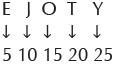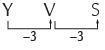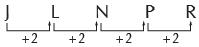Courses

# Olympiad Test : Alphabet Test And Logical Sequence - 2

## 20 Questions MCQ Test Science Olympiad for Class 5 | Olympiad Test : Alphabet Test And Logical Sequence - 2

Description
This mock test of Olympiad Test : Alphabet Test And Logical Sequence - 2 for Class 5 helps you for every Class 5 entrance exam. This contains 20 Multiple Choice Questions for Class 5 Olympiad Test : Alphabet Test And Logical Sequence - 2 (mcq) to study with solutions a complete question bank. The solved questions answers in this Olympiad Test : Alphabet Test And Logical Sequence - 2 quiz give you a good mix of easy questions and tough questions. Class 5 students definitely take this Olympiad Test : Alphabet Test And Logical Sequence - 2 exercise for a better result in the exam. You can find other Olympiad Test : Alphabet Test And Logical Sequence - 2 extra questions, long questions & short questions for Class 5 on EduRev as well by searching above.
QUESTION: 1

### ​In the question, some letters are given, which are numbered 1,2,3,4 and 5. Below are given four options containing combinations of these numbers. Find the combination of numbers so that the letters arranged accordingly form a meaningful word. E H R S O 1 2 3 4 5

Solution:

The meaningful word is ‘HORSE’ so correct combination numbers is 25341.

QUESTION: 2

### If in the word ‘DISTURBANCE’ the first letter is interchanged with the last letter, second letter is interchanged with the tenth letter and so on; which letter would come after the letter ‘R’ in the newly formed word?

Solution:

DISTURBANCE By interchanging we get ECNABRUTSID. so, after letter R, U will come.

QUESTION: 3

### Study the given letter series and answer the questions that follow: H D Y S M W N B Q P O C R T B L Z V E G U F Q. Which is the only letter that occurs twice?

Solution:

After studying the given letter series carefully, we come to know that B occurs twice.

QUESTION: 4

Study the given letter series and answer the questions that follow:
H D Y S M W N B Q P O C R T B L Z V E G U F
Q. Which three letters have the same distance as they have in the alphabetical order although they have changed paces?

Solution:

In the given series as well as in the alphabet, there are 12 letters between L and Y and 5 Letters between F and L.

QUESTION: 5

Study the given letter series and answer the questions that follow:
H D Y S M W N B Q P O C R T B L Z V E G U F
Q. Which two neighbours in the given arrangement are the farthest in the alphabetical order?

Solution:

Between D and Y there are 20 letters, so they are the farthest in the alphabetical order.

QUESTION: 6

Study the given letter series and answer the questions that follow:
H D Y S M W N B Q P O C R T B L Z V E G U F
Which letter has the same neighbours as in the alphabetical order although they have changed places?

Solution:

P has O and Q as neighbours in the given series and English alphabet.

QUESTION: 7

In these questions a group of letters is given, which are numbered 1,2,3,4 and 5. Below are given four alternatives containing combination of these numbers. Select the combination of numbers, which form a meaningful word.
​U R I F T
1 2 3 4 5

Solution:

The meaningful word is ‘FRUIT’ so
correct combination of numbers is
42135.

QUESTION: 8

In these questions a group of letters is given, which are numbered 1,2,3,4 and 5. Below are given four alternatives containing combination of these numbers. Select the combination of numbers, which form a meaningful word.
​A L B T E
1 2 3 4 5

Solution:

The meaningful word is ‘TABLE’ so correct combination of numbers is 41325.

QUESTION: 9

In these questions a group of letters is given, which are numbered 1,2,3,4 and 5. Below are given four alternatives containing combination of these numbers. Select the combination of numbers, which form a meaningful word.
​R E D I P
1 2 3 4 5

Solution:

The meaningful word is ‘PRIDE’ so correct combination of numbers is 51432.

QUESTION: 10

In these questions a group of letters is given, which are numbered 1,2,3,4 and 5. Below are given four alternatives containing combination of these numbers. Select the combination of numbers, which form a meaningful word.
​A C E T R
1 2 3 4 5

Solution:

The meaningful word is ‘TRACE’ so correct combination of numbers is 45123.

QUESTION: 11

​Each of the following questions is based on the following alphabet series.
A B C D E______________Z
Q. Which letter is seventh to the right of the eighteenth letter from the right end of the alphabet?

Solution:

Seventh to the right of eighteen letter from the right end means 18–7 =11th letter. From VQLGB, Q’ s position is 10th from right. So P will be the 11th from right.

QUESTION: 12

​Each of the following questions is based on the following alphabet series.
A B C D E______________Z
Q. If the letters of the given alphabet interchange positions, so that A takes the place of Z and Z takes the place of A; B takes the place of Yand Y takes the place of B and so on. What will be the seventieth letter from the right

Solution:

The new alphabet series will be Z Y X W V U T S R Q P O N M L K J I H G F E D C B A So, counting from right, we get ‘Q’ as seventeenth letter of alphabet. It can be solved by E J O T Y formula also. By reversing the order of alphabet. and counting from right means the normal order of alphabet.So seventeenth letter will be Q. O’s position is 15th inQUESTION: 13

​Each of the following questions is based on the following alphabet series.
A B C D E______________Z
Q. If the second half of the given alphabet is written in reverse order, which letter should be fourth to the left of twelfth letter from the right.

Solution:

As per the question, the new alphabet series is A B C D E F G H I J K L M Z Y X W V U T S R Q P O N Counting from A, the twelfth letter from the right is Y. Fourth to the left of twelfth means 12 + 4 = 16th letter from the right ie. K.

QUESTION: 14

Each of the following questions is based on the following alphabet series.
A B C D E______________Z
Q. Number of letters skipped in between adjacent letters in the series decreases by three. Which of the following series observes this rule?

Solution:QUESTION: 15

​Each of the following questions is based on the following alphabet series.
A B C D E______________Z
Q. The letters skipped in between the adjacent letters in the series are followed by equal space. Which of the following series observes this rule.

Solution:So, J L N P R series observes the
rule.

QUESTION: 16

​In each of following questions, arrange the words in a meaningful and logical sequence and select the appropriate sequence from the options given below for each of the group of words.
Q.1. Mother 2. Child 3. Milk 4. Cry 5. Smile

Solution:

Child ⟶ Cry ⟶ Mother ⟶ Milk ⟶Smile

QUESTION: 17

​In each of following questions, arrange the words in a meaningful and logical sequence and select the appropriate sequence from the options given below for each of the group of words.
1. Uttar Pradesh 2. Universe 3. World 4. India 5. Bulandshahr

Solution:

Universe ⟶ World ⟶ India ⟶ Uttar Pradesh ⟶ Bulandshahr

QUESTION: 18

​In each of following questions, arrange the words in a meaningful and logical sequence and select the appropriate sequence from the options given below for each of the group of words.
(i) Plant (ii) Fruit (iii) Seed (iv) Flower

Solution:

Seed ⟶ Plant ⟶ Flower ⟶ Fruit

QUESTION: 19

​In each of following questions, arrange the words in a meaningful and logical sequence and select the appropriate sequence from the options given below for each of the group of words.
​(i) Cooking
(ii) Vegetable Market
(iii) Lady finger
(iv) Dish
(V) Cutting

Solution:

Vegetable Market ⟶ Lady finger ⟶ Cutting ⟶ Cooking ⟶ Dish

QUESTION: 20

​In each of following questions, arrange the words in a meaningful and logical sequence and select the appropriate sequence from the options given below for each of the group of words.
(i) House (ii) Town (iii) State (iv) District (v) Street

Solution:

State ⟶ District ⟶ Town ⟶ Street ⟶ House